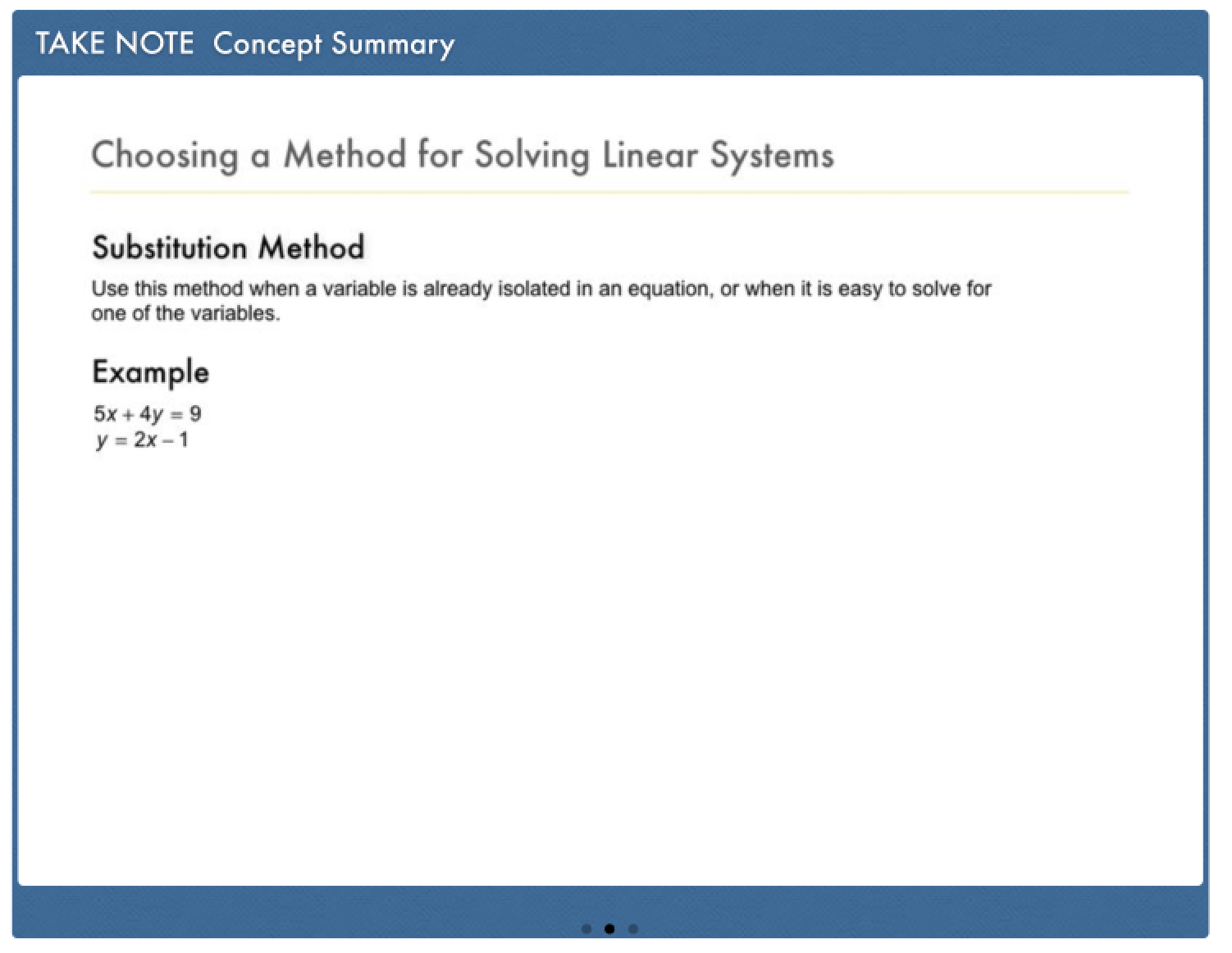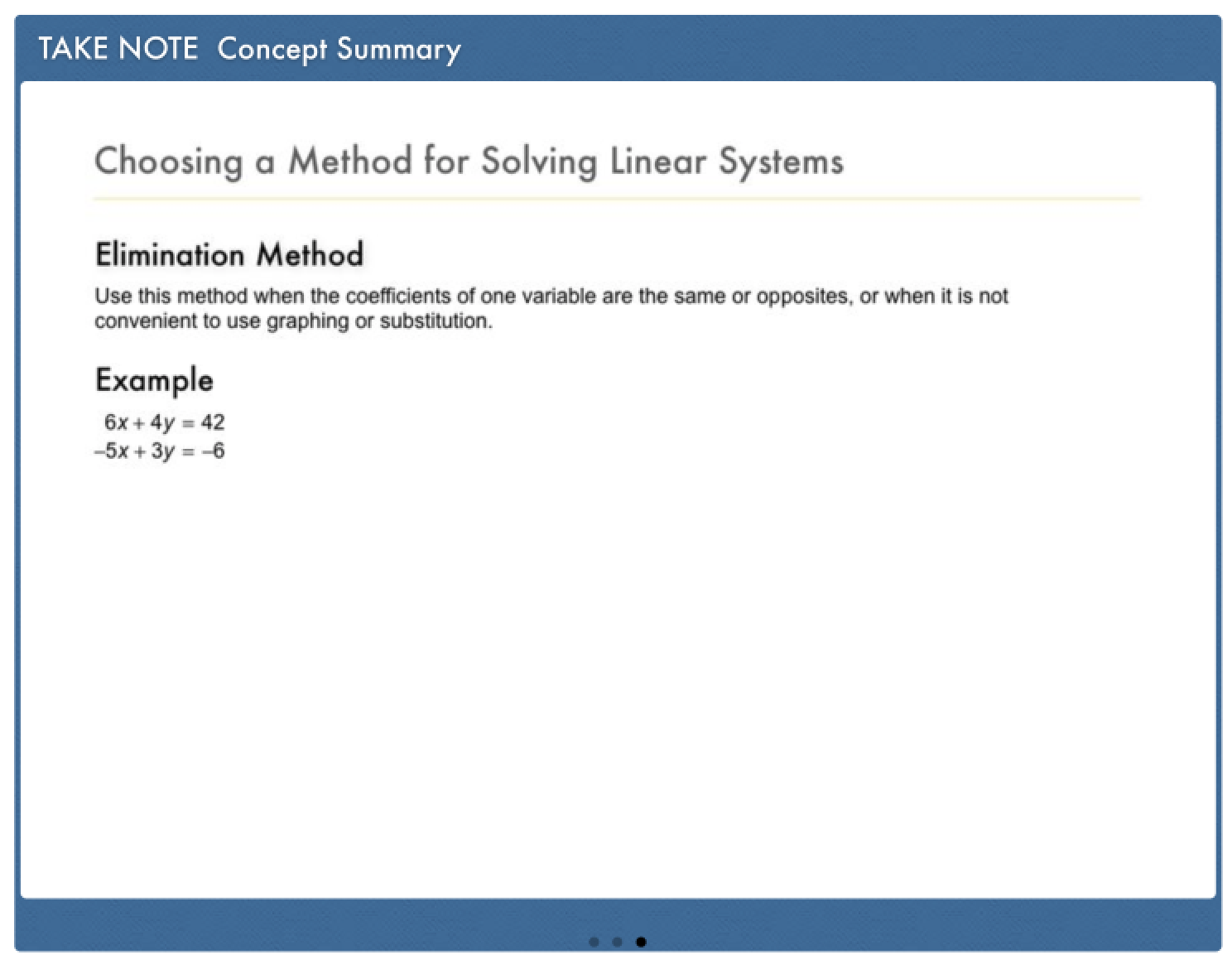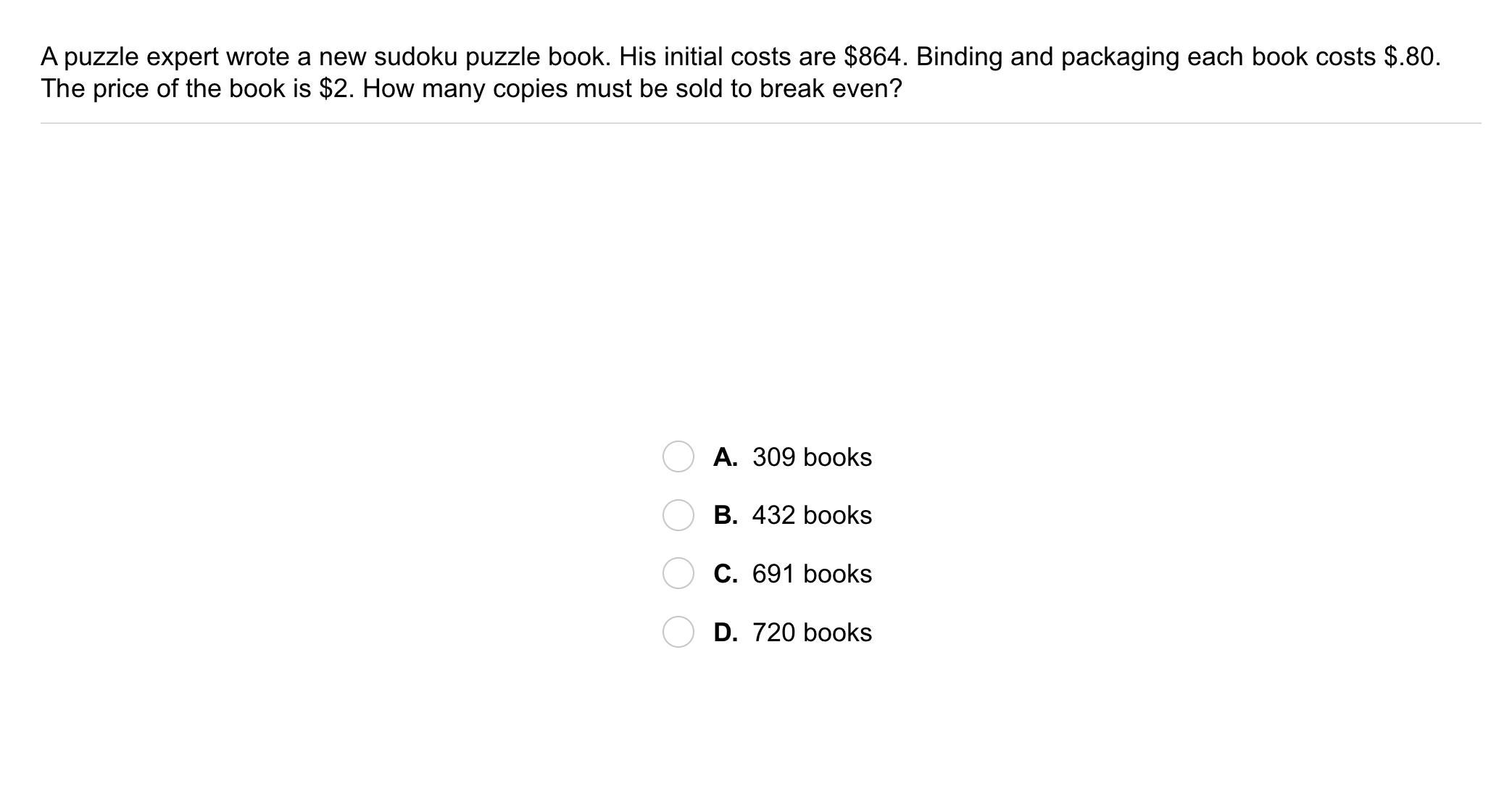Algebra 1 6-4 Guided Practice: Applications of Linear Systems
starstarstarstarstarstarstarstarstarstar
by Matthew Richardson
| 10 Questions1
1
10
Solve It! The taller candle burns at a rate of 1.15 in. per hour. The shorter candle burns at a rate of 0.75 in. per hour. After how many hours will they be the same height?
3
5
7
82
3
4
5
2
10
Take Note: Describe how to determine if a system of linear equations is a good candidate to solve using the graphing method.
3
10
Take Note: Describe how to determine if a system of linear equations is a good candidate to solve using the substitution method.
4
10
Take Note: Describe how to determine if a system of linear equations is a good candidate to solve using the elimination method.
5
10
Take Note: Define break-even point.6
6
10
Problem 1 Got It? A puzzle expert wrote a new sudoku puzzle book. His initial costs are \$864. Binding and packaging each book costs \$.80. The price of the book is \$2. How many copies must be sold to break even?
A
B
C
D7
7
10
Problem 2 Got It? The zoo has two water tanks that are leaking. One tank contains 10 gal of water and is leaking at a constant rate of 2 gal/h. The second tank contains 6 gal of water and is leaking at a constant rate of 4 gal/h. When will the tanks have the same amount of water? Explain.
A
B
C
D8
8
10
Problem 3 Got It?
A
B
C
D9
9
10
Problem 3 Got It?
A
B
C
D10
10
10
Take Note: Summarize the content of this lesson.